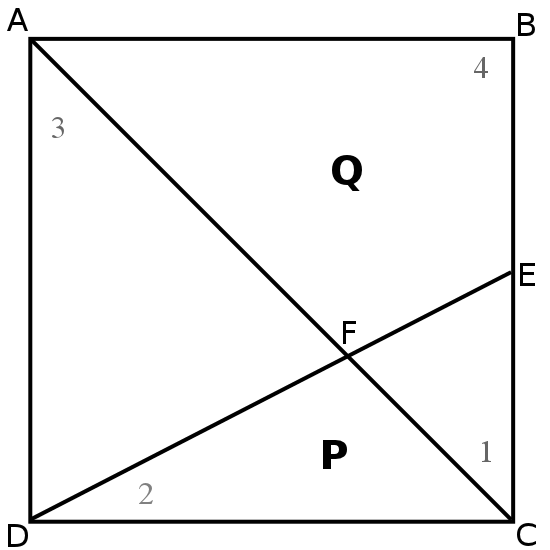You may also likeSome(?) of the Parts

A circle touches the lines OA, OB and AB where OA and OB are perpendicular. Show that the diameter of the circle is equal to the perimeter of the triangleTriangle Midpoints

You are only given the three midpoints of the sides of a triangle. How can you construct the original triangle?Fermat's Poser

Find the point whose sum of distances from the vertices (corners) of a given triangle is a minimum.

Cut-up Square

Age 14 to 16 Short Challenge Level:First, we label the corners of the square, the midpoint, and the point at which the two lines intersect $A$, $B$, $C$, $D$, $E$ and$F$ respectively. We also label the four triangles created within the square as 1, 2, 3 and 4.

Call the area of triangle a.

Angle $AFD$ is equal to angle $EFC$ as they are vertically opposite, and angle $FAD$ is equal to angle $FCD$ as they are alternate. So, triangles 1 and 3 are similar. As $E$ marks the midpoint of a side of the square, length $CE$ is half the value of length $AD$.

This means that the area of triangle 3 is equal to 4a, as its base and height are double that of triangle 1.

It also means that length $AF$ is equal to twice the value of length $FC$. If we let $AF$ be the base of triangle 3 and $FC$ be the base of triangle 2, then these triangles have the same height (equal to the perpendicular distance from $D$ to the line $AF$) so the area of triangle 2 is equal to half that of triangle 3 - that is, 2a.

As line $AC$ is a diagonal, the combined areas of triangles 2 and 3 is equal to half the area of the square. 4a + 2a = 6a, so the square has a total area of 12a. The combined area of triangle 1 and triangle 4 is then also 6a. As we have defined the area of triangle 1 as a, we then know that triangle 4 has an area of 5a.

As the ratio of $P:Q$ is simply the ratio area of triangle 2:area of triangle 4, we then know that this ratio is 2a:5a=2:5.

This problem is taken from the UKMT Mathematical Challenges.
You can find more short problems, arranged by curriculum topic, in our short problems collection.